# The Logic of Observation and Belief Revision in Scientific Communities

## Abstract

Scientists collect evidence in order to confirm or falsify scientific theories. Unfortunately, scientific evidence may sometimes be false or deceiving and as a consequence lead individuals to believe in a false theory. By interaction between scientists, such false beliefs may spread through the entire community. There is currently a debate about the effect of various network configurations on the epistemic reliability of scientific communities (e.g. Zollman 2010 and Rosenstock et al. 2017). To contribute to this debate from a logical perspective, this paper introduces an epistemic logical framework of observation, interaction and belief revision in scientific communities. The presented sound and complete system provides the formal tools for qualitative analysis of the social dynamics of scientific inquiry. Furthermore, this paper includes detailed suggestions for future applications of the framework.

This is a preview of subscription content, access via your institution.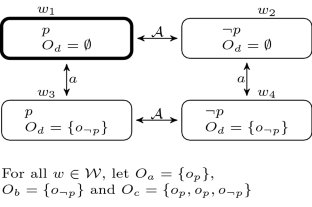Fig. 1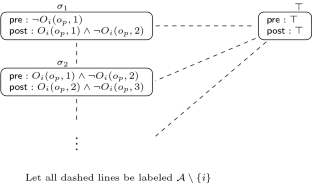Fig. 2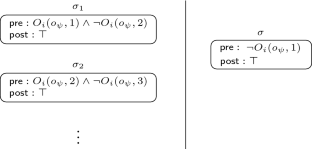Fig. 3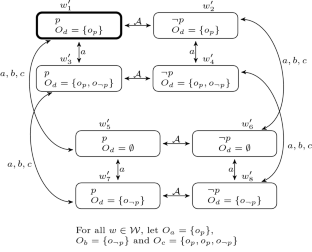Fig. 4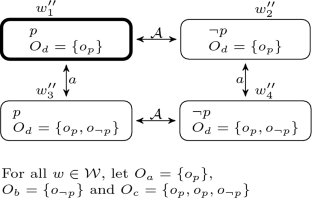Fig. 5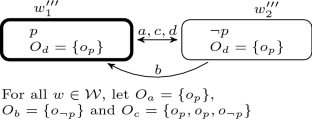Fig. 6
1. 1.

In the social sciences, collection of evidence may also be done through experience. We focus here on the collection of evidence through observation.

2. 2.

For example, suppose some agent a learns that some other agent b believes that some medicine A is effective, while agent a knows that agent b herself has observed that medicine A is not effective. Agent a might then reason about agent b’s knowledge of the observations of agent b’s peers, which may have been reason for agent b to believe that A is in fact effective. See Sect. 3.4 for an illustration of this situation in our framework.

3. 3.

For results in this direction we refer to Baltag et al. (1998), Hintikka (1962).

4. 4.

In the ensuing, the construct $$o_{\psi }$$ is interpreted as a single observation. Alternatively, $$o_{\psi }$$ may be interpreted as a conclusion on a collection of observations.

5. 5.

Note that our formal constructs for observations are structurally simpler than the evidence terms in existing evidence logics and justification logics, cf. Artemov (2008), Baltag et al. (2012).

6. 6.

With a finite limit, the disjunction $$\bigvee _{n\le L}O_i(o_p,n)$$ (appearing in the action model for revising beliefs, Definition 16) is a finite formula. Furthermore, by imposing a limit on n, the action model for observing (Definition 12) will necessarily be finite. To maintain flexibility, the exact value of L is open.

7. 7.

While $$K_i$$ expresses a much stronger notion of knowledge, satisfying the $${{\mathsf {S}}}{{\mathsf {5}}}$$ properties, $$\square _i$$ is much weaker and satisfies the $${{\mathsf {S}}}{{\mathsf {4}}}$$ properties. We refer the reader to Baltag and Smets (2008a) for more details about the differences and use of these epistemic attitudes.

8. 8.

Note that Stalnaker (2006) defined a notion of belief based on knowledge; in particular he interprets belief as the “epistemic possibility of knowledge” (Stalnaker 2006, 180). Our definition of belief in this paper can be interpreted in exactly the same spirit.

9. 9.

The restriction that N be reflexive and symmetric is chosen in accordance to existing studies on the relation between epistemic reliability and network structure (e.g. Zollman 2010). Therefore, the logic presented in this paper only captures the class of models with reflexive and symmetric neighborhood mappings N. When investigating different network restrictions, Definition 2 may be changed accordingly.

10. 10.

That $$<_i$$ is $$\sim _i$$-locally conversed well-founded means that given any $$w\in {\mathcal {W}}$$, every non-empty subset of $$\{v\in {\mathcal {W}}\mid w\sim _i v\}$$ has a $$<_i$$-maximal element $$v^*$$ such that there is no $$v'\in \{v\in {\mathcal {W}}\mid w\sim _i v\}$$ such that $$v^*<v'$$.

11. 11.

To be more explicit: the valuation $$O_i$$ maps a world $$w\in {\mathcal {W}}$$ to an element of $${\mathcal {P}}(\langle {\mathscr {O}},m_{L}\rangle )$$, where $$m_{L}$$ is a special multiplicity function that maps every $$o_{\psi }\in {\mathscr {O}}$$ to the limit L. See Appendix 1 for details on multisets.

12. 12.

In alterations of the framework, one could argue for a weaker condition for upgrading that is based on defeasible knowledge.

13. 13.

One could additionally propose a contraction action (cf. Liu et al. 2014) for the case where agent i knows that exactly $$\theta$$ of agent i’s neighbors have observed p more often than $$\lnot p$$ and exactly $$1-\theta$$ of agent i’s neighbors have observed $$\lnot p$$ more often than p. A contraction action would make the p-worlds and $$\lnot p$$-worlds equi-plausible. The current precondition says that in this case, the agent does not upgrade her beliefs.

14. 14.

In a more complex framework, one could define belief upgrade as a private action. As this is beyond the scope of this paper, we opt for the simpler public version.

15. 15.

Cognitive diversity of a scientific community entails that as many different possible theories are investigated by at least one individual.

16. 16.

There are many theories that try to account for this delay, such as Oreskes (1999). More generally, Kuhn’s paradigm shift accounts for such collective failures as well (Kuhn 1962).

17. 17.

To obtain a close comparison between models of $${\mathsf {MEOL}}$$ and Zollman’s models, it must be noted that Zollman’s models are in particular suitable for studying variations of a particular informational dilemma, often referred to as a bandit problem. In such a scenario, agents are faced with a dilemma to gain information by performing a combination of different actions or to choose the one action with the highest expected payoff (for detailed explanation, see Zollman 2007). If an agent facing a bandit problem chooses to optimize her payoffs, she will not gain information about the suboptimal actions. As a consequence, her knowledge development will rely on the choices and communication of other agents. Translating this scenario into our models in a similar way as Zollman did may be done as follows. Imagine an agent a that wants to know which of two medicines is more successful. Let $$p_1$$ be the expression that some medicine A is successful with a 70% chance (which is in fact true); $$q_1$$ express that medicine B is successful with a 60% chance; and $$q_2$$ that medicine B is successful with a 80% chance (which is in fact also true). Suppose agent a believes $$p_1$$ and $$q_1$$. Note that this implies that agent a believes that medicine A is more successful than medicine B. A bandit problem will be formed by restricting the agents’ possible observations by protocols or by strengthening the precondition of the action model $$o_{\psi }+_i$$ (see Sect. 4.2 for details protocols) to only allow for observations involving medicine A when an agent believes medicine A to be superior to medicine B. That is, because in the sketched scenario, agent a can then only find out that $$q_2$$ is true if other scientists observe $$q_2$$ and communicate this with her. Note that this scenario involves probabilistic propositions that are semantically related (e.g., $$q_1\wedge q_2\rightarrow \bot$$). Such interrelations may be formalized in standard $${\mathsf {DEL}}$$, but it may be more clear to use variations of $${\mathsf {DEL}}$$ that do include probabilities, such as presented in Baltag and Smets (2008b), van Benthem et al. (2009), Kooi (2003). As Zollman’s analyses are based on probabilistic propositions, a close comparison to his conclusion is quite unnatural to non-probabilistic logical frameworks. Rather, we encourage to use our logic for a more general comparison on the structural properties of belief formation in scientific communities.

18. 18.

And for technical reasons, the modeler should also specify a limit L. The limit should be high enough to let the agents in the model make as least as many observations as needed for the case under study.

19. 19.

Our framework may also be used to study the social phenomenon pluralistic ignorance (see Hendricks and Hansen 2016) in a scientific context. An often discussed problem of the phenomenon is that agents only see other agents’ expressed belief (i.e., their behavior) and have no access to inner believe or private deliberation that motivated this behavior (Liu et al. 2014; Rendsvig 2014). The same applies to models of $${\mathsf {MEOL}}$$, with ‘expressed’ observation sets on the one hand and ‘inner’ belief on the other, so a comparison may be informative.

## References

1. Artemov, S. N. (2008). The logic of justification. Review of Symbolic Logic, 1(4), 477–513.

2. Bala, V., & Goyal, S. (1998). Learning from neighbours. Review of Economic Studies, 65(3), 565–621.

3. Baltag, A., Christoff, Z., Hansen, J. U., & Smets, S. (2013). Logical models of informational cascades. In J. van Benthem, & F. Liu (Eds.), Proceedings of the Tsinghua logic conference: Logic across the university: Foundations and applications (Vol. 47, pp. 405–432). London: College Publications.

4. Baltag, A., Christoff, Z., Rendsvig, R. K., & Smets, S. (2018). Dynamic epistemic logics of diffusion and prediction in social networks. Studia Logica. https://doi.org/10.1007/s11225-018-9804-x.

5. Baltag, A., Moss, L. S., & Solecki, S. (1998). The logic of common knowledge, public announcement, and private suspicions. In I. Gilboa (Ed.), Proceedings of the 7th conference on Theoretical aspects of rationality and knowledge (TARK 1998) (pp. 43–56). Morgan Kaufmann Publishers Inc.

6. Baltag, A., & Renne, B. (2016). Dynamic epistemic logic. In E. N. Zalta (Ed.), The Stanford Encyclopedia of Philosophy. Stanford: Metaphysics Research Lab, Stanford University. (Winter 2016 edition).

7. Baltag, A., Renne, B., & Smets, S. (2012). The logic of justified belief change, soft evidence and defeasible knowledge. In L. Ong, & R. de Queiroz (Eds.), Proceedings of the 19th workshop on logic, language, information and computation, volume 7456 of lecture notes in computer science (pp. 168–190). Berlin: Springer.

8. Baltag, A., & Smets, S. (2008a). A qualitative theory of dynamic interactive belief revision. In G. Bonanno, W. van der Hoek, & M. Wooldridge (Eds.), Logic and games 3 (pp. 13–60). Amsterdam: Amsterdam University Press.

9. Baltag, A., & Smets, S. (2008b). Probabilistic dynamic belief revision. Synthese, 165(2), 165–179.

10. Baltag, A., van Ditmarsch, H. P., & Moss, L. S. (2008). Epistemic logic and information update. In P. Adriaans, & J. van Benthem (Eds.), Philosophy of information. Cambridge: MIT Press.

11. van Benthem, J. (2007). Dynamic logic for belief revision. Journal of Applied Non-Classical Logics, 17(2), 129–155.

12. van Benthem, J. (2011). Logical dynamics of information and interaction. Cambridge: Cambridge University Press.

13. van Benthem, J., Gerbrandy, J., Hoshi, T., & Pacuit, E. (2009). Merging frameworks for interaction. Journal of Philosophical Logic, 38(5), 491–526.

14. van Benthem, J., Gerbrandy, J., & Kooi, B. (2009). Dynamic update with probabilities. Studia Logica, 93(1), 67–96.

15. van Benthem, J., & Pacuit, E. (2011). Dynamic logics of evidence-based beliefs. Studia Logica, 99(1–3), 61–92.

16. Blackburn, P., de Rijke, M., & Venema, Y. (2001). Modal logic. Cambridge: Cambridge University Press.

17. Borg, A., Frey, D., Šešelja, D., & Straßer, C. (2017). Examining network effects in an argumentative agent-based model of scientific inquiry. In A. Baltag, J. Seligman, & T. Yamada (Eds.), Proceedings of the logic, rationality, and interaction: 6th international workshop, LORI 2017. Lecture notes in computer science (pp. 391–406) Berlin: Springer.

18. Christoff, Z. (2016). Dynamic logics of networks: Information flow and the spreak of opinion. PhD thesis. University of Amsterdam, ILLC.

19. van Ditmarsch, H. P., & Kooi, B. (2008). Semantic results for ontic and epistemic change. In G. Bonanno, W. van der Hoek, & M. Wooldridge (Eds.), Logic and the foundations of game and decision theory (LOFT 7) (pp. 87–117). Amsterdam: Amsterdam University Press.

20. van Ditmarsch, H. P., van der Hoek, W., & Kooi, B. (2008). Dynamic epistemic logic, volume 337 of synthese library. Berlin: Springer.

21. Frenkel, V. Y. (1979). On the history of the Einstein-de Haas effect. Soviet Physics Uspekhi, 22(7), 580–587.

22. Gerbrandy, J., & Groeneveld, W. (1997). Reasoning about information change. Journal of Logic, Language and Information, 6(2), 147–169.

23. Hendricks, V. F., & Hansen, P. G. (2016). Infostorms, why do we ’like’? Explaining individual behavior on the social net (2nd ed.). Berlin: Springer.

24. Hintikka, J. (1962). Knowledge and belief: An introduction to the logic of the two notions. Ithaca: Cornell University Press.

25. Kitcher, P. (1990). The division of cognitive labor. Journal of Philosophy, 87(1), 5–22.

26. Kooi, B. P. (2003). Probabilistic dynamic epistemic logic. Journal of Logic, Language and Information, 12(4), 381–408.

27. Kuhn, T. S. (1962). The structure of scientific revolutions. Chicago: University of Chicago Press.

28. Liu, F., Seligman, J., & Girard, P. (2014). Logical dynamics of belief change in the community. Synthese, 191(11), 2403–2431.

29. Miller, B., & Steyvers M. (2011). The wisdom of crowds with communication. Proceedings of the Annual Meeting of the Cognitive Science Society, 33, 1292–1297.

30. Niiniluoto, I. (2011). Revising beliefs towards the truth. Erkenntnis, 75(2), 165–181.

31. Oreskes, N. (1999). The rejection of continental drift: Theory and method in American earth science. Oxford: Oxford University Press.

32. Palmer, E. (1954). Investigation of the gastric mucosa spirochetes of the human. Gastroenterology, 27(2), 218–220.

33. Pedersen, D. B., & Hendricks, V. F. (2014). Science bubbles. Philosophy and Technology, 27(4), 503–518.

34. Rendsvig, R. K. (2014). Pluralistic ignorance in the bystander effect: Informational dynamics of unresponsive witnesses in situations calling for intervention. Synthese, 191(11), 2471–2498.

35. Rendsvig, R. K. (2015). Model transformers for dynamical systems of dynamic epistemic logic. In W. van der Hoek, W. Holliday, & W. Wang (Eds.), Logic, rationality, and interaction. LORI 2015. Lecture notes in computer science (Vol. 9394, pp. 316-327). Heidelberg, Berlin: Springer.

36. Renne, B. (2012). Multi-agent justification logic: Communication and evidence elimination. Synthese, 185(S1), 43–82.

37. Rosenstock, S., Bruner, J., & O’Connor, C. (2017). In epistemic networks, is less really more? Philosophy of Science, 84(2), 234–252.

38. Stalnaker, R. (2006). On logics of knowledge and belief. Philosophical Studies, 128(1), 169–199.

39. Wegener, A. (1912). Die Herausbildung der Großformen der Erdrinde (Kontinente und Ozeane), auf geophysikalischer Grundlage. Geologische Rundschau, 3(4), 276–292.

40. Weisberg, M., & Muldoon, R. (2007). Epistemic landscapes and the division of cognitive labor. Philoosphy of Science, 76(2), 225–252.

41. Zollman, K. J. (2007). The communication structure of epistemic communities. Philosophy of Science, 74(5), 574–587.

42. Zollman, K. J. (2010). The epistemic benefit of transient diversity. Erkenntnis, 72(1), 17–35.

43. Zollman, K. J. (2013). Network epistemology: Communication in epistemic communities. Philosophy Compass, 8(1), 15–27.

Download references

## Acknowledgements

The research of Hanna Sofie van Lee is financed by the Carlsberg Foundation. We would like to thank Alexandru Baltag, Vincent F. Hendricks, Rasmus K. Rendsvig and two anonymous reviewers for valuable comments.

## Author information

Authors

### Corresponding author

Correspondence to Hanna Sofie van Lee.

## Appendices

### 1. Multisets

Here, some definitions on multisets are presented. This may be helpful to understand the nature of observation sets $$O_i$$.

### Definition 1

(Multiset) A multisetA is a pair $$\langle {\mathscr {A}},f\rangle$$ where $${\mathscr {A}}$$ is a regular set and $$f:{\mathscr {A}}\rightarrow {\mathbb {N}}$$ a function, giving the multiplicity of each $$a\in {\mathscr {A}}$$. That is, f will tell how often a is included in the multiset. Unlike for regular sets, in multisets it holds that $$\{a,a\}\ne \{a\}$$.

### Definition 2

(Sub-multiset) Suppose $$A=\langle {\mathscr {A}},f\rangle$$ and $$B=\langle {\mathscr {B}},g\rangle$$ are multisets. A is a sub-multiset of B, $$A\subseteq B$$, if for all $$a\in {\mathscr {A}}$$ it holds that $$f(a)\le g(a)$$ (and < for proper sub-multiset $$A\subset B$$).

### Definition 3

(Multi-powerset) Suppose $$A=\langle {\mathscr {A}},f\rangle$$ is a multiset. The multi-powerset of A, $${\mathcal {P}}(\langle {\mathscr {A}},f\rangle )$$, is defined by taking all sub-multiplicities.

Consider as an example multiset $$A=\{a,b,b\}$$. Its power set is given by $${\{\emptyset ,\{a\},\{b\},\{a,b\},\{a,b\},\{b,b\},\{a,b,b\}\}}$$.

### Definition 4

(Support) Suppose $$A=\langle {\mathscr {A}},f\rangle$$ is a multiset. The subset $${\mathbf {A}}$$ of $${\mathscr {A}}$$ is called the support of A if $$a\in {\mathbf {A}}$$ iff $$f(a)>0$$.

### Definition 5

(Positive multiset) Suppose $$A=\langle {\mathscr {A}},f\rangle$$ is a multiset. The positive multiset$$A^{+}$$ of A is defined as $$\langle {\mathbf {A}},f^{+}\rangle$$ such that $${\mathbf {A}}$$ is the support of A and $$f^{+}(a){:=}f(a)$$.

For construction of the canonical model in the completeness proof of $${\mathsf {MEOL}}^s$$ (see Appendix 2), define the union of two multisets:

### Definition 6

(Union multisets) Suppose $${\mathbf {A}}=\langle A,f\rangle$$ and $${\mathbf {B}}=\langle A,g\rangle$$ are multisets. Their union, $${\mathbf {A}}\cup {\mathbf {B}}$$, is $${\mathbf {C}}=\langle A,h\rangle$$ where for all $$a\in A$$ it holds that $$h(a)=\mathsf {max}(f(a),g(a))$$.

### Theorem 1

(Soundness of $${\mathsf {MEOL}}^s$$) $${\mathsf {MEOL}}^s$$ is sound: for all$$\varphi \in {\mathscr {F}}^s$$ and all$$\varPhi \subseteq {\mathscr {F}}^s$$ it holds that

\begin{aligned} \hbox {if }\varPhi \vdash \varphi \hbox { then }\varPhi \models \varphi \end{aligned}

### Proof

We prove soundness by induction on the length k of derivation. For the base case, $$\varphi$$ must be an axiom of $${\mathsf {MEOL}}^s$$. As most axioms of $${\mathsf {MEOL}}^s$$ (Definition 9) match with the semantic conditions (Definition 4), it follows directly for those axioms that $$\models \varphi$$. Additionally, Multiset follows semantically by Property 2 and Neighborhood holds semantically by truth definition of $$N_ij$$ (Definition 7). For the induction step, suppose that for $$\varphi$$ derived at step k, it holds that if $$\varPhi \vdash \varphi$$ then $$\varPhi \models \varphi$$. Consider $$\gamma$$ that is derived by applying one of the rules at the $$k+1$$-step. For each rule it follows by the induction hypothesis and the truth definitions of $$\rightarrow$$, $$\square _i$$ and $$K_i$$ (Definition 7) that respectively $$\models \gamma$$, $$\models \square _i\varphi$$ and $$\models K_i\varphi$$.□

### Theorem 2

(Completeness of $${\mathsf {MEOL}}^s$$) $${\mathsf {MEOL}}^s$$ is strongly complete. That is, for all$$\varphi \in {\mathscr {F}}^s$$ and all$$\varPhi \subseteq {\mathscr {F}}^s$$ it holds that

\begin{aligned} \hbox {if } \varPhi \models \varphi \hbox { then } \varPhi \vdash \varphi \end{aligned}

### Proof

We prove by way of a canonical model construction. Given sets of atomic propostions $$\varPhi$$, agents $${\mathcal {A}}$$, and a limit L, define the canonical model $$\varOmega {:=}({\mathcal {W}}^{\varOmega },{\mathcal {V}},O_i,\le _i,\sim _i)$$ by setting

\begin{aligned} W^{\varOmega }&{:=}\{\varGamma \subseteq {\mathscr {F}}^s\mid \varGamma \text { is maximal consistent}\}\\ {\mathcal {V}}(\varGamma )&{:=}\{p\in \varPhi \mid p\in \varGamma \}\\ O_i(\varGamma )&{:=}\bigcup _{O_i(o_{\psi },n)\in \varGamma }(o_{\psi },n)\\ \varGamma \le _i\varDelta&\text { iff }\{\varphi \mid \square _i\varphi \in \varGamma \}\subseteq \varDelta \\ \varGamma \sim _i\varDelta&\text { iff }\{\varphi \mid K_i\varphi \in \varGamma \}\subseteq \varDelta \end{aligned}

We will first prove that $$\varOmega$$ is a model for $${\mathsf {MEOL}}^s$$, by showing that it satisfies all semantic properties. We use standard methods to show that $$\le _i$$ is a preorder and that $$\sim _i$$ is an equivalence relation.

To prove indefeasibility we show $$\varGamma \le _i\varDelta \rightarrow \varGamma \sim _i\varDelta$$ for all $$i\in {\mathcal {A}}$$. Suppose not. Then there exists $$i,\varGamma ,\varDelta$$ such that $$\varGamma \le _i\varDelta$$ while $$\varGamma \not \sim _i\varDelta$$. This implies that there is a $$\varphi$$ such that $$K_i\varphi \in \varGamma$$ while $$\varphi \not \in \varDelta$$. By the axiom Indefeasibility, it follows that $$\square _i\varphi \in \varGamma$$. Since $$\varGamma \le _i\varDelta$$ this implies $$\varphi \in \varDelta$$. By contradiction we conclude that indefeasibility holds.

To prove local connectedness we show $$\varGamma \sim _i\varDelta \rightarrow (\varGamma \le _i\varDelta \vee \varDelta \le _i\varGamma )$$ for all $$i\in {\mathcal {A}}$$. Suppose not. Then there exists $$i,\varGamma ,\varDelta$$ such that $$\varGamma \sim _i\varDelta$$ while $$\varGamma \not \le _i\varDelta$$ and $$\varDelta \not \le _i\varGamma$$. This implies that there are $$\gamma ,\varphi$$ such that $$\square _i\gamma \in \varGamma$$$$(*)$$ while $$\gamma \not \in \varDelta$$, and $$\square _i \varphi \in \varDelta$$ while $$\varphi \not \in \varGamma$$. As $$\gamma \not \in \varDelta$$: $$\lnot \gamma \in \varDelta$$ and hence $$\lnot K_i\varphi \in \varGamma$$. As $$\varphi \not \in \varGamma$$: $$\lnot \varphi \in \varGamma$$ and hence $$\lnot K_i\gamma \in \varGamma$$ (because $$\sim _i$$ is reflexive). Hence, $$\lnot (K_i\varphi \vee K_i\gamma )\in \varGamma$$. By axiom Local Connectedness, it follows that either i) $$\lnot K_i(\varphi \vee \square _i\gamma )\in \varGamma$$ or ii) $$\lnot K_i(\gamma \vee \square _i\varphi )\in \varGamma$$. In case i), then $$K_i(\lnot \varphi \wedge \lnot \square _i\gamma )\in \varGamma$$ which implies $$\lnot \square _i\gamma \in \varGamma$$. This contradicts to $$(*)$$. In case ii), $$K_i(\lnot \gamma \wedge \lnot \square _i\varphi )\in \varGamma$$ which implies $$\lnot \gamma \in \varGamma$$. So $$\lnot \square _i\gamma \in \varGamma$$ (because $$\le _i$$ is reflexive). This contradicts to $$(*)$$. Thus, local connectedness holds in the model.

Now we will prove observation introspection: $$\varGamma \sim _i\varDelta \rightarrow O_i(\varGamma )=O_i(\varDelta )$$ for all $$i\in {\mathcal {A}}$$. Suppose not, then there exists $$i,\varGamma ,\varDelta$$ such that $$\varGamma \sim _i\varDelta$$ although there is a pair $$(o_{\psi },n)$$ such that either $$(o_{\psi },n)\subseteq O_i(\varGamma )$$ but $$(o_{\psi },n)\not \subseteq O_i(\varDelta )$$, or $$(o_{\psi },n)\not \subseteq O_i(\varGamma )$$ and $${(o_{\psi },n)\subseteq O_i(\varDelta )}$$. Suppose the former holds. Then $$O_i(o_{\psi },n)\in \varGamma$$. By axiom Observation Introspection: $$K_iO_i(o_{\psi },n)\in \varGamma$$. Since $$\varGamma \sim _i\varDelta$$ it follows that $$O_i(o_{\psi },n)\in \varDelta$$ and thus $$(o_{\psi },n)\subseteq O_i(\varDelta )$$. By contradiction and similar argument for the other case, we conclude that observation introspection holds.

To finish the proof, we need to show that the Truth Lemma holds: for each $$\varGamma \in W^{\varOmega }$$ and each $$\varphi \in {\mathscr {F}}$$, we have $$\varphi \in \varGamma$$ iff $$\varGamma \models _{\varOmega }\varphi$$. We prove this by induction on the construction of $$\varphi$$. For the base cases, i) $$\varphi =\top$$ or ii) $$\varphi =p$$. Since $$\varGamma$$ is a maximal consistent set, $$\top \in \varGamma$$ is always true, so by truth definition, it follows that $$\varGamma \models _{\varOmega }\top$$. Likewise, $$p\in \varGamma$$ iff $$p\in {\mathcal {V}}(\varGamma )$$ iff $$\varGamma \models _{\varOmega }p$$. Then for the induction steps, assume the induction hypothesis: for all $$\gamma ,\chi$$ of less complexity than $$\varphi$$ the Truth Lemma holds. For case i) $$\varphi =\lnot \gamma$$ it holds that $$\lnot \gamma \in \varGamma$$ iff $$\gamma \not \in \varGamma$$ iff $$\varGamma \nvDash _{\varOmega }\gamma$$ iff $$\varGamma \models _{\varOmega }\lnot \gamma$$. For case ii) $$\varphi =\gamma \wedge \chi$$, $$\gamma \wedge \chi \in \varGamma$$ iff $$\gamma \in \varGamma$$ and $$\chi \in \varGamma$$ iff $$\varGamma \models _{\varOmega }\gamma$$ and $$\varGamma \models _{\varOmega }\chi$$ iff $$\varGamma \models \gamma \wedge \chi$$. For case iii) $$\varphi =O_i(o_{\psi },n)$$ it holds that $$O_i(o_{\psi },n)\in \varGamma$$ iff $$(o_{\psi },n)\subseteq O_i(\varGamma )$$ iff $$\varGamma \models _{\varOmega } O_i(o_{\psi },n)$$. We continue with case iv) $$\varphi =N_ij$$: $$N_ij\in \varGamma$$ iff $$\varGamma \vdash N_ij$$ iff $$N_ij$$ (by Neighborhood axiom) iff $$\varGamma \models _{\varOmega } N_ij$$. For case v) $$\varphi =\square _i\gamma$$, $$\square _i\gamma \in \varGamma$$ iff for all $$\varDelta \ge _i\varGamma$$ it holds that $$\gamma \in \varDelta$$ (by definition of canonical model) iff $$\varDelta \models _{\varOmega }\gamma$$ for all $$\varDelta \ge _i\varGamma$$ iff $$\varGamma \models _{\varOmega }\square _i\gamma$$ (by truth definition). Finally for case vii) $$\varphi =K_i\gamma$$, $$K_i\gamma \in \varGamma$$ iff $$\gamma \in \varGamma$$ for all $$\varDelta \sim _i\varGamma$$ iff $$\varDelta \models _{\varOmega }\gamma$$ for all $$\varDelta \sim _i\varGamma$$ iff $$\varGamma \models _{\varOmega } K_i\gamma$$.□

### Theorem 3

(Soundness and completeness of $${\mathsf {MEOL}}$$) For all$$\varphi \in {\mathscr {F}}$$ it holds that:

\begin{aligned} \models \varphi \hbox { iff }\vdash \varphi \end{aligned}

### Proof

The proof system of $${\mathsf {MEOL}}$$ contains reduction axioms such that for each $$\varphi \in {\mathscr {F}}$$, there is a reduced $$\varphi ^{\dagger }\in {\mathscr {F}}^s$$ such that

\begin{aligned} \vdash \varphi \leftrightarrow \varphi ^{\dagger } \end{aligned}

We will prove that the reduction axioms hold also semantically in the models of $${\mathsf {MEOL}}$$:

\begin{aligned} \models \varphi \leftrightarrow \varphi ^{\dagger } \end{aligned}

We will demonstrate the proof only for some of the more complex formulas, as the techniques are standard. We show Observation Dynamics: $$\models [\alpha ,\sigma ]O_i(o_{\psi },n)\leftrightarrow {({\mathsf {pre}}_{\sigma }\rightarrow ({\mathsf {post}}_{\sigma }\rightarrow O_i(o_{\psi },n)))}$$. First from left to right: assume for an arbitrary $$({\mathcal {M}},w)$$ that $${({\mathcal {M}},w)\models [\alpha ,\sigma ]O_i(o_{\psi },n)}$$. We know that either $$({\mathcal {M}},w)\nvDash {\mathsf {pre}}_{\sigma }$$ or $$({\mathcal {M}},w)\models {\mathsf {pre}}_{\sigma }$$. Suppose the former holds, then it directly follows that $$({\mathcal {M}},w)\models {\mathsf {pre}}_{\sigma }\rightarrow {({\mathsf {post}}_{\sigma }\rightarrow O_i(o_{\psi },n))}$$. Now suppose the latter holds. Assume for contradiction that $$({\mathcal {M}},w)\nvDash {\mathsf {pre}}_{\sigma }\rightarrow {({\mathsf {post}}_{\sigma }\rightarrow O_i(o_{\psi },n))}$$. Then $$({\mathcal {M}},w)\models {\mathsf {pre}}_{\sigma } \wedge {\mathsf {post}}_{\sigma } \wedge \lnot O_i(o_{\psi },n))$$ ($$\star$$). We know by truth definition of $${\mathsf {MEOL}}$$ that since $$({\mathcal {M}},w)\models {\mathsf {pre}}_{\sigma }$$ and $$({\mathcal {M}},w)\models [\alpha ,\sigma ]O_i(o_{\psi },n)$$, it holds that $${\mathcal {M}}\otimes \alpha ,(w,\sigma )\models O_i(o_{\psi },n)$$. Hence $$(o_{\psi },n)\in O_i^{\alpha }(w,\sigma )$$. This implies by definition of $$O_i^{\alpha }$$ that either (i) $$({\mathcal {M}},w)\models {\mathsf {post}}_{\sigma }\rightarrow O_i(o_{\psi },n)$$, which contradicts to ($$\star$$), or (ii) not $$({\mathcal {M}},w)\nvDash {\mathsf {post}}_{\sigma }\rightarrow O_i(o_{\psi },n)$$, and $$({\mathcal {M}},w)\models O_i(o_{\psi },n)$$. This again contradicts to ($$\star$$). Hence, $$({\mathcal {M}},w)\models {\mathsf {pre}}_{\sigma }\rightarrow ({\mathsf {post}}_{\sigma }\rightarrow O_i(o_{\psi },n))$$. Now from right to left, by contraposition: assume that $$({\mathcal {M}},w)\nvDash [\alpha ,\sigma ]O_i(o_{\psi },n)$$. Then $$({\mathcal {M}},w)\models {\mathsf {pre}}_{\sigma }$$ and not $${\mathcal {M}}\otimes \alpha ,(w,\sigma )\models O_i(o_{\psi },n)$$. This implies that $$(w,\sigma )\not \in [\![O_i(o_{\psi },n)]\!]^{\alpha }$$. Now assume for contradiction that $$({\mathcal {M}},w)\models {\mathsf {post}}(\sigma )\rightarrow O_i(o_{\psi },n)$$. By $$({\mathcal {M}},w)\models {\mathsf {pre}}_{\sigma }$$ and by definition of $$O_i^{\alpha }$$, $$(o_{\psi },n)\in O_i^{\alpha }(w,\sigma )$$. Contradiction. Hence, $$({\mathcal {M}},w)\models \lnot ({\mathsf {post}}_{\sigma }\rightarrow O_i(o_{\psi },n)$$. And therefore, $$({\mathcal {M}},w)\nvDash {\mathsf {pre}}_{\sigma }\rightarrow ({\mathsf {post}}_{\sigma }\rightarrow O_i(o_{\psi },n))$$.

We continue proving the Knowledge Dynamics I: $$\models [\alpha ,\sigma ]\square _i\varphi \leftrightarrow ({\mathsf {pre}}_{\sigma }\rightarrow \square _i[\alpha ,\sigma ]\varphi )$$ for $$\alpha \in \{o_{\psi }+_i,O_i(o_{\psi },n)!_i,\lnot O_i(o_{\psi },1)!_i\}$$ for every $$\psi \in \Psi$$. From left to right: suppose $${({\mathcal {M}},w)\models [\alpha ,\sigma ]\square _i\varphi }$$. Then $${\mathcal {M}}\otimes \alpha ,(w,\sigma )\models \square _i\varphi$$. This means that for all $$(v,\sigma )\ge _i (w,\sigma )$$: $${\mathcal {M}}\otimes \alpha ,(w,\sigma )\models \varphi$$. By definition of $${\mathcal {M}}\otimes \alpha$$, for all $$v\ge _i w$$: $$({\mathcal {M}},v)\models [\alpha ,\sigma ]\varphi$$. Hence $$({\mathcal {M}},w)\models \square _i[\alpha ,\sigma ]\varphi$$, which implies $$({\mathcal {M}},w)\models {\mathsf {pre}}_{\sigma }\rightarrow \square _i[\alpha ,\sigma ]\varphi$$. From right to left, suppose $$({\mathcal {M}},w)\models {\mathsf {pre}}_{\sigma }\rightarrow \square _i[\alpha ,\sigma ]\varphi$$. Now if $$({\mathcal {M}},w)\models {\mathsf {pre}}_{\sigma }$$, then $$({\mathcal {M}},w)\models \square _i[\alpha ,\sigma ]\varphi$$. By same steps as above, but inversed, it follows that $${\mathcal {M}}\otimes \alpha ,(w,\sigma )\models \square _i\varphi$$ which implies that $$({\mathcal {M}},w)\models [\alpha ,\sigma ]\square _i\varphi$$. If $$({\mathcal {M}},w)\nvDash {\mathsf {pre}}_{\sigma }$$, then we follow the usual steps.

Next, we prove Knowledge Dynamics II: $$\models [\alpha ,\sigma ]\square _i\varphi \leftrightarrow ({\mathsf {pre}}_{\sigma }\rightarrow (\square _i(\gamma \rightarrow [\alpha ,\sigma ]\varphi )\wedge \lnot \gamma \rightarrow (\square _i[\alpha ,\sigma ]\varphi \wedge K_i(\gamma \rightarrow [\alpha ,\sigma ]\varphi ))))$$. First, from left to right. Suppose $$({\mathcal {M}},w)\models [\alpha ,\sigma ]\square _i\varphi$$ ($$*$$). As before, when $$({\mathcal {M}},w)\nvDash {\mathsf {pre}}_{\sigma }$$ then we’re done. Suppose $$({\mathcal {M}},w)\models {\mathsf {pre}}_{\sigma }$$ and for contradiction, suppose $$({\mathcal {M}},w)\nvDash (\square _i(\gamma \rightarrow [\alpha ,\sigma ]\varphi )\wedge \lnot \gamma \rightarrow (\square _i[\alpha ,\sigma ]\varphi \wedge K_i(\gamma \rightarrow [\alpha ,\sigma ]\varphi )))$$. Suppose $$({\mathcal {M}},w)\nvDash \square _i(\gamma \rightarrow [\alpha ,\sigma ]\varphi )$$. Then there is a v such that $$w\le _i v$$ and $$({\mathcal {M}},v)\models \gamma$$ while $$({\mathcal {M}},v)\nvDash [\alpha ,\sigma ]\varphi$$, thus $${\mathcal {M}}\otimes \alpha ,(v,\sigma )\nvDash \varphi$$. However, by our assumption ($$*$$), we know that $${\mathcal {M}}\otimes \alpha ,(w,\sigma )\models \square _i\varphi$$. Note that $$(w,\sigma )\le _i(v,\sigma )$$ (by definition of $$\le _i^{\alpha }$$), so $${{\mathcal {M}}\otimes \alpha ,(v,\sigma )\models \varphi }$$. Contradiction. Now suppose $$({\mathcal {M}},w)\nvDash \lnot \gamma \rightarrow (\square _i[\alpha ,\sigma ]\varphi \wedge {K_i(\gamma \rightarrow [\alpha ,\sigma ]\varphi ))}$$. Then $${({\mathcal {M}},w)\models \lnot \gamma }$$ but $$({\mathcal {M}},w)\nvDash \square _i[\alpha ,\sigma ]\varphi$$ or $$({\mathcal {M}},w)\nvDash K_i(\gamma \rightarrow [\alpha ,\sigma ]\varphi ))$$. In the first case, there must be some $$v\ge _i w$$ such that $$({\mathcal {M}},v)\nvDash [\alpha ,\sigma ]\varphi$$ and thus $${\mathcal {M}}\otimes \alpha ,(v,\sigma )\nvDash \varphi$$. Note that again $$(w,\sigma )\le _i(v,\sigma )$$, which implies together with ($$*$$) that $${\mathcal {M}}\otimes \alpha ,(v,\sigma )\models \varphi$$. Contradiction. In the second case, there must be a $$v\sim _i w$$ such that $$({\mathcal {M}},v)\models \gamma$$ and $$({\mathcal {M}},v)\nvDash [\alpha ,\sigma ]\varphi$$. Hence $${\mathcal {M}}\otimes \alpha ,(v,\sigma )\nvDash \varphi$$. As $$({\mathcal {M}},w)\models \lnot \gamma$$ and $$({\mathcal {M}},v)\models \gamma$$ and $$w\sim _i v$$, it follows that $$(w,\sigma )\le _i(v,\sigma )$$. In the same way as before, this leads to a contradiction. Hence $$({\mathcal {M}},w)\models (\square _i(\gamma \rightarrow [\alpha ,\sigma ]\varphi )\wedge \lnot \gamma \rightarrow (\square _i[\alpha ,\sigma ]\varphi \wedge K_i(\gamma \rightarrow [\alpha ,\sigma ]\varphi )))$$. For right to left, suppose the right side of the equivalence relation holds at $$({\mathcal {M}},w)$$. For purpose of contradiction, suppose $$({\mathcal {M}},w)\nvDash [\alpha ,\sigma ]\square _i\varphi$$. This implies $$({\mathcal {M}},w)\models {\mathsf {pre}}_{\sigma }$$ and $${\mathcal {M}}\otimes \alpha ,(w,\sigma )\nvDash \square _i\varphi$$. So there is a $$(v,\sigma )$$ such that $$(w,\sigma )\le _i(v,\sigma )$$ while $${\mathcal {M}}\otimes \alpha ,(v,\sigma )\nvDash \varphi$$. By definition of $$(w,\sigma )\le _i(v,\sigma )$$, it follows that either i) $$\gamma \not \in {\mathcal {V}}(w)$$ and $$\gamma \in {\mathcal {V}}(v)$$ and $$w\sim _i v$$, or ii) $$\gamma \not \in {\mathcal {V}}(w)$$ and $$w\sim _i v$$, or iii) $$\gamma \in {\mathcal {V}}(v)$$ and $$w\sim _i v$$. In case of i), then $$({\mathcal {M}},w)\models \lnot \gamma$$, so $$({\mathcal {M}},w)\models K_i(\gamma \rightarrow [\alpha ,\sigma ]\varphi )$$. Since $$w\sim _i v$$ and $$({\mathcal {M}},v)\models \gamma$$, this implies that $$({\mathcal {M}},w)\models [\alpha ,\sigma ]\varphi$$ and thus that $${\mathcal {M}}\otimes \alpha ,(v,\sigma )\models \varphi$$. Contradiction. In case of ii), then $$w\le _i v$$ and thus $$({\mathcal {M}},v)\models [\alpha ,\sigma ]\varphi$$. Again this implies that $${\mathcal {M}}\otimes \alpha ,(v,\sigma )\models \varphi$$. Contradiction. In case of iii), it also follows that $$({\mathcal {M}},v)\models [\alpha ,\sigma ]\varphi$$ and hence that $${\mathcal {M}}\otimes \alpha ,(v,\sigma )\models \varphi$$. So we get a contradiction once again. This proves that $$({\mathcal {M}},w)\models [\alpha ,\sigma ]\square _i\varphi$$ and thereby finishes the proof for Knowledge Dynamics II.

Finally, we prove Knowledge Dynamics III: $$\models [\alpha ,\sigma ]K_i\varphi \leftrightarrow ({\mathsf {pre}}_{\sigma }\rightarrow K_i[\alpha ,\sigma ]\varphi )$$. Left to right: suppose $$({\mathcal {M}},w)\models [\alpha ,\sigma ]K_i\varphi$$, so $${\mathcal {M}}\otimes \alpha ,(w,\sigma )\models K_i\varphi$$. By definition of $${\mathcal {M}}[ \alpha ,\sigma ]$$, this implies that for all $$(v,\sigma )$$ such that $$(w,\sigma )\sim _i (v,\sigma )$$ it holds that $${\mathcal {M}}\otimes \alpha ,(v,\sigma )\models \varphi$$. Then, for all v such that $$w\sim _i v$$ it holds that $$({\mathcal {M}},w)\models [\alpha ,\sigma ]\varphi$$ and thus $${({\mathcal {M}},w)\models K_i[\alpha ,\sigma ]\varphi }$$. From right to left: suppose $$({\mathcal {M}},w)\models {\mathsf {pre}}_{\sigma }\rightarrow K_i[\alpha ,\sigma ]\varphi$$. If $$({\mathcal {M}},w)\models {\mathsf {pre}}_{\sigma }$$, then $${({\mathcal {M}},w)\models K_i[\alpha ,\sigma ]\varphi }$$. Thus, for all v such that $$w\sim _i v$$ it holds that $$({\mathcal {M}},w)\models [\alpha ,\sigma ]\varphi$$. So for all $$(v,\sigma )$$ s.t. $$(w,\sigma )\sim _i (v,\sigma )$$: $${\mathcal {M}}\otimes \alpha ,(v,\sigma )\models \varphi$$, which implies $${\mathcal {M}}\otimes \alpha ,(w,\sigma )\models K_i\varphi$$. Therefore, $$({\mathcal {M}},w)\models [\alpha ,\sigma ]K_i\varphi$$. If $$({\mathcal {M}},w)\nvDash {\mathsf {pre}}_{\sigma }$$, then the proof continues as before.

By Theorems 1 and 2, this implies soundness and completeness of $${\mathsf {MEOL}}$$.□

## Rights and permissions

Reprints and Permissions